Orginal Article

# NON-ORDINARY STATE-BASED PERIDYNAMIC THERMAL-VISCOPLASTIC MODEL AND ITS APPLICATION

Wang Han, Huang Dan*, Xu Yepeng, Liu Yiming

College of Mechanics and Materials , Hohai University , Nanjing 211100, China

Abstract

A three-dimensional non-local thermo-visco-plastic solid model considering the strain rate effect, plastic hardening, thermal softening and fracture characteristics of materials, together with corresponding non-local spatial integral-type numerical method, have been proposed under the configuration of the recently developed non-ordinary state-based peridynamic (NOSB-PD) theory, and the model and numerical method have been employed to analyze the high-rate thermal-viscoplastic deformation and failure behavior of metallic materials and components under impact loads. The validity of the proposed model and algorithms was established through simulating the three-dimensional classical Kalthoff-Winkler impact experiment and comparing the numerical results including the cracking initiation time and orientation, crack propagation path and propagation speed, and the distribution of temperature and equivalent stresses in the target with experimental observations and available numerical results in literature. The proposed model and method were further applied to simulate the deformation and failure mechanism of double-notched metallic plates subjected to impact loads with different impacting velocities. Numerical results show that the present model inherits the advantages of both peridynamics and classical thermo-visco-plastic models, and is able to describe the whole elastic and plastic deformation and crack propagation processes qualitatively as well as quantitatively. Moreover, the effect of impact velocity on crack initiation time, crack propagation path and crack propagation speed were investigated. When subjected to impact load with lower impacting velocity, the crack initiation time of the target plate will be later (until no crack propagation appears when impacting velocity is lower than some value), and both the crack propagation speed and the peak temperature in the target plate will decrease.

Keywords： impact failure ; thermo-visco-plasticity ; non-ordinary stated-based peridynamics ; crack propagation ; non-local model ; Kalthoff-Winkler experiment

0

Wang Han, Huang Dan, Xu Yepeng, Liu Yiming. NON-ORDINARY STATE-BASED PERIDYNAMIC THERMAL-VISCOPLASTIC MODEL AND ITS APPLICATION[J]. Acta Mechanica Sinica, 2018, 50(4): 810-819 https://doi.org/10.6052/0459-1879-18-113

## 1 非常规态型近场动力学热黏塑性模型

### 1.1 近场动力学基本方程

$Y¯〈ξ〉=y'-y=ξ+η$

$ρ(x)ü(x,t)=b(x,t)+∫Hx‍(T¯[x,t]〈x'₈$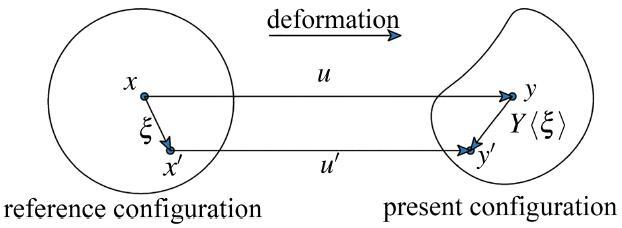Fig.1   Interaction between material points

### 1.2 NOSB-PD热黏塑性本构模型

$K[x,t]=∫Hx‍ω(|ξ|)(ξ⊗ξ)dVx'$

$F=∂y(x,t)∂x$

$F[x,t]=∫Hx‍ω(|ξ|)(Y¯〈ξ〉⊗ξ)dVx'K-1$

$Ḟ=∫Hx‍ω(|ξ|)(v¯⊗ξ)dVx'K-1$

$L=∂v∂x=ḞF-1$

$D=12(L+LT),W=12(L-LT)$

$D=De+Dvp+DT$

$De=B:τ∇$

$Dvp=3ε̅̇2σ̅S̅$

$S̅=S-α$

$S$为偏应力张量

$S=τ-13τ:I$

$ε̅̇=ε̅̇0σ̅g(ε̅,T)m$

$g(ε̅,T)=σ01+ε̅ε0n[1-δ0(eT-T0κ-1)]$

$DT=αṪI$

$Ṫ=χε̅̇σ̅ρCp$

$σ̅=(32S:S)12$

$ε̅=∫0t‍(23Dvp:Dvp)12dt$

$P=τF-T$

$T¯〈ξ〉=ω〈ξ〉PK-1ξ$

### 1.3 非常规态型近场动力学破坏模型

$σ=J-1τ$

$μ(t)=1,σr<[σ]0,others$

$ω(|ξ|)=ω0(|ξ|)μ$

### 1.4 数值离散与求解

$ρ(xi)ü(xi,t)=b(xi,t)+∑j=1m‍(T¯[xi,t]〈xj-xi〉-T¯[xj,t]〈xi-xj〉)ΔVxj,i=1,2,…,N$

$τn+1=τn+τ̇n+1Δt$

$τ̇n+1=τ∇n+1+Wn+1⋅τn+τnWn+1T$

$τ∇=C:(D-Dvp-DT)=C:(D-3ε̅̇2σ̅S-αṪI)$

$Δε̅n+1=O(Δt3)+ε̅̇nΔt+Δt2∂ε̅̇∂ε̅nε̅̇n+1+∂ε̅̇∂σ̅nσ̅̇n+1+∂ε̅̇∂TnṪn+1$

$ε̅̇n+1=O(Δt2)+ε̅̇n+Δt∂ε̅̇∂ε̅nε̅̇n+1+∂ε̅̇∂σ̅nσ̅̇n+1+∂ε̅̇∂TnṪn+1$

$σ̅̇=32σ̅S:τ∇$

$Pn+1=C:pn,pn=32Snσ̅n$

$ε̅̇n+1=ε̅̇n1+ξn+1+ξn+1(1+ξn+1)Hn+1Pn+1:Dn+1$

$Hn+1=3μ-∂ε̅̇/∂ε̅∂ε̅̇/∂σ̅n-∂ε̅̇/∂T∂ε̅̇/∂σ̅nαχσ̅nρCpξn+1=Δt∂ε̅̇∂σ̅nHn+1$

$τ∇n+1=Cn+1tan:Dn+1-ε̅̇n1+ξn+1Pn+1+3λαχσ̅n+1ρCpI$

$Cn+1tan=C-ξn+1Hn+1(1+ξn+1)⋅Pn+1⊗Pn+1+(3λ+2μ)αχσ̅n+1ρCpI⊗Pn+1$

## 2 模型验证

Kalthoff-Winkler冲击试验[31-32]是经典的金属动态断裂问题, 在该试验中用刚性弹丸冲击带有对称预置裂纹的金属靶板, 观测裂纹的扩展过程, 包括起裂时间、扩展方向和路径等. 在高速冲击试验过程中, 可观测到刚性弹丸撞击靶板后产生的应力波经板底反射后与裂尖相互作用; 裂纹扩展路径与冲击速度有关, 且裂纹扩展区温度效应明显. 作为一种复杂的冲击作用下热黏塑性变形与破坏过程, Kalthoff-Winkler 试验的仿真已成为检验本构模型和数值方法的经典考题. Li等[2-3,33]、Belytschko等和Song等 均采用不同的数值方法对该试验进行了数值建模与过程仿真.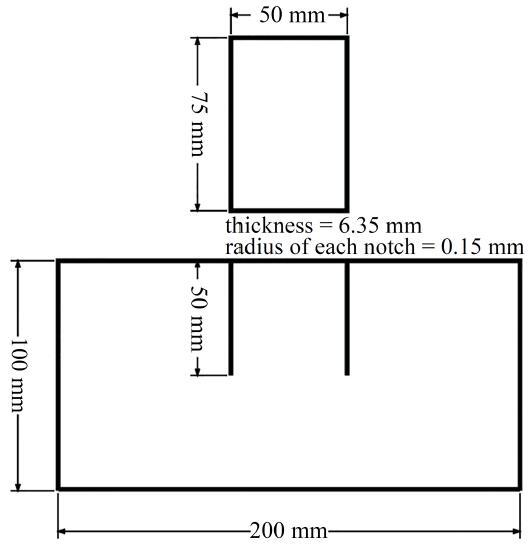Fig.2   Model for Kalthoff-Winkler test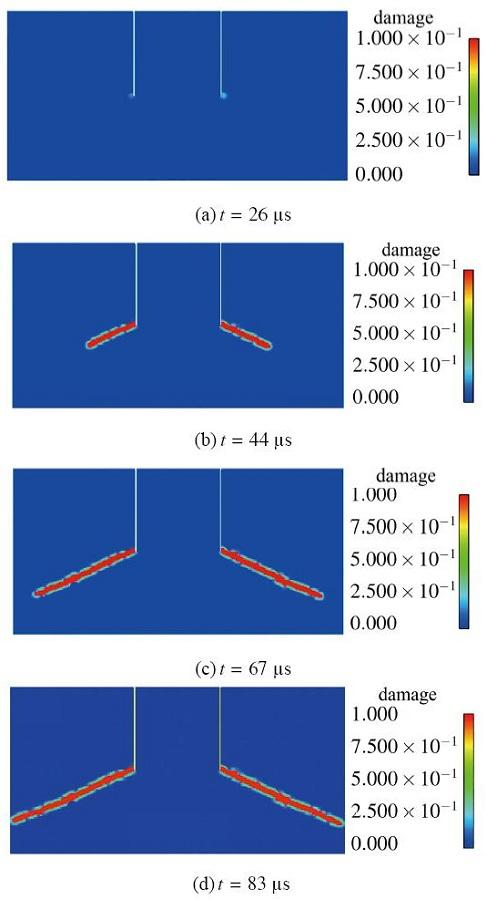Fig.3   Crack propagation of the Kalthoff-Winkler test

$vi=yi-yi-lti-ti-l=yi-yi-lΔt$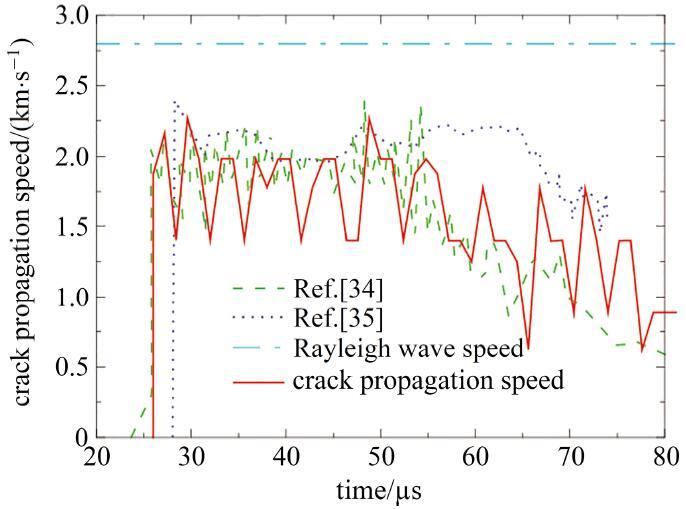Fig.4   Crack propagation speed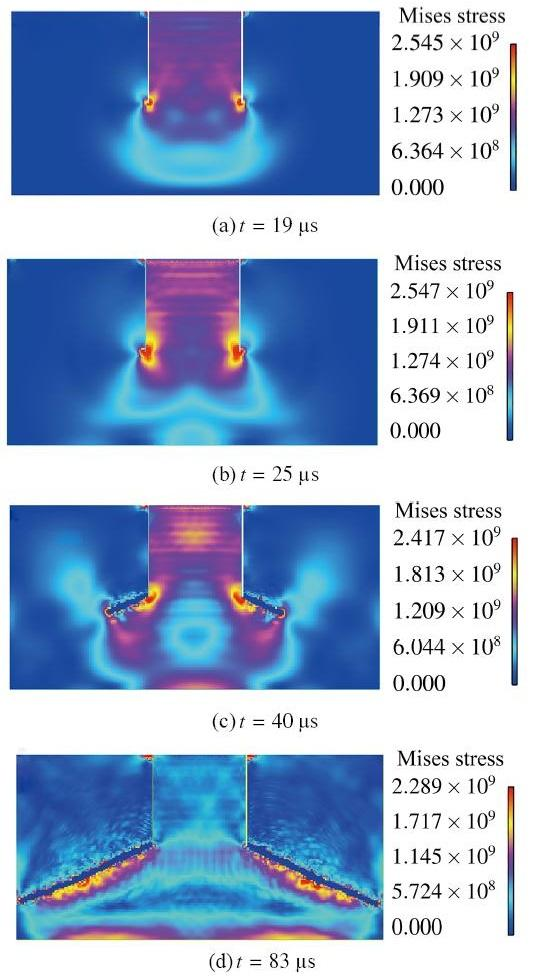Fig.5   Mises stress contours

## 3 热黏塑性近场动力学模型的应用

### 3.1 裂纹起裂、贯通时间以及裂纹扩展角度

Table 1   Crack propagation angle

ImpactingCrack initiationCutting-throughAngle/
velocity/(m$⋅$s$-1$)time/μstime/μs(°)
268367.997
288669.693
299271.118
309375.362
3680.166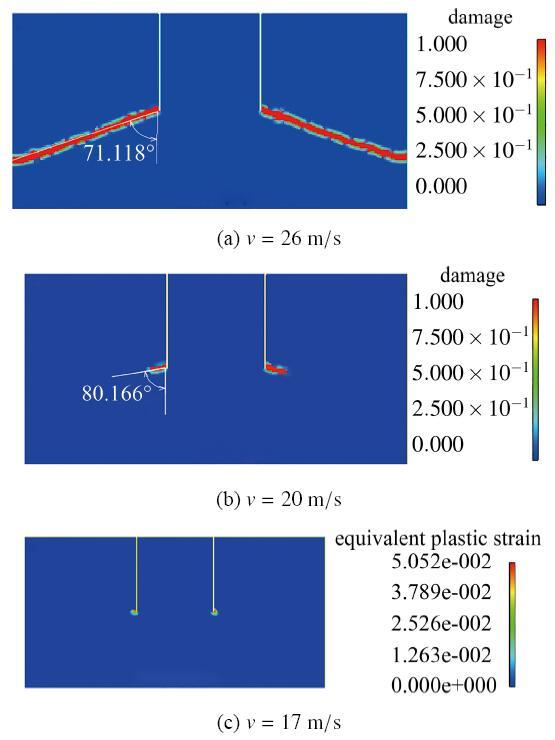Fig.6   Crack initiation and propagation

### 3.2 裂纹扩展速度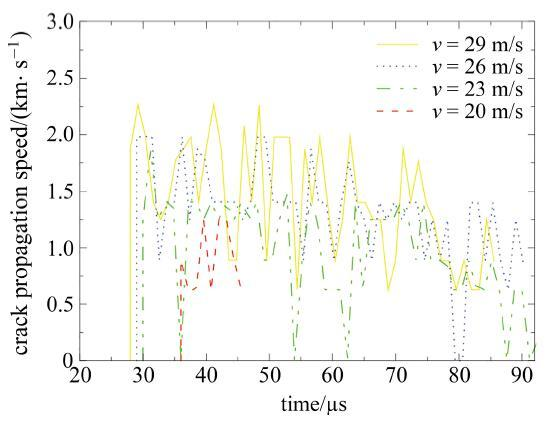Fig.7   Crack propagation speed in different cases

### 3.3 靶板的最高温度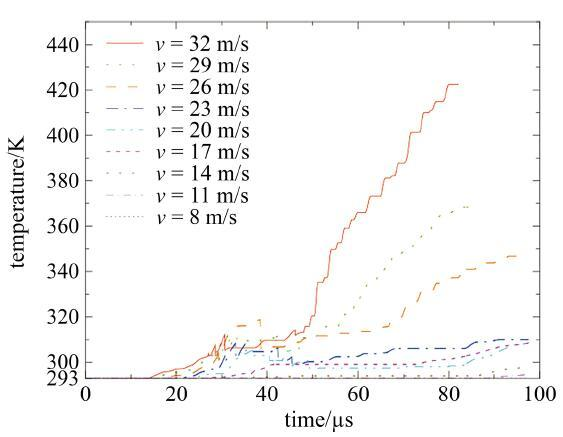Fig.8   The peak temperature in the target plate during the impact

Table 2   The peak temperature of the target plate

Impacting velocity/(m$⋅$s$-1$)Maximum temperature/K
422.5
369.5
348.4
310.1
308.4
308.6
298.1
294.7
293.0

## 4 结 论

(1)本文构建的非常规态型近场动力学热黏塑性本构模型以及相应的非局部数值计算体系, 能以较高精度和计算效率模拟金属材料和结构在冲击载荷作用下的变形和破坏过程, 得到裂纹起裂时间、扩展方向, 定量描述整个裂纹扩展过程以及冲击过程中的剪切温升, 反映率效应.

(2)通过对冲击速度32 m/s工况下的Kalthoff-Winkler试验进行数值模拟, 得到了初始裂纹的扩展角度、扩展速度与裂纹开裂过程中等效应力的分布. 模拟结果所得裂纹扩展角度与实验结果高度吻合, 所得裂纹扩展速度的大小与变化趋势与文献结果一致. 模拟中可再现破坏的物理机制: 应力波经靶板反射后与裂尖相互作用, 最终形成破坏.

(3)应用本文模型分析不同冲击速度下经典Kalthoff-Winkler冲击试验中裂纹的扩展角度、扩展速度, 以及冲击过程中靶板剪切带的温度变化. 随着冲击速度下降, 裂纹的扩展角度(与竖直方向的夹角)将不断增大, 起裂越晚, 裂纹扩展速度峰值越低, 冲击过程中靶板破坏区域(剪切带)的温度峰值越低, 裂纹完全扩展至边缘所需时间越长. 冲击速度低于17 m/s时, 靶板将仅产生塑性变形而不会产生裂纹扩展, 此时所能达到的温度峰值高于冲击速度为20 m/s的温度峰值. 当冲击速度为低于8 m/s 时, 靶板中不产生温度变化, 仅发生弹性变形.

(4)上述结果验证了本文构建的非常规态型近场动力学热黏塑性固体模型在分析金属类材料冲击响应方面的可行性与有效性. 在此基础上, 可望在后续工作中针对复杂条件下金属类材料和结构的三维冲击损伤破坏问题开展定量规律研究和全过程数值模拟, 并为类似问题的深入研究提供参考.

The authors have declared that no competing interests exist.

## 参考文献 原文顺序 文献年度倒序 文中引用次数倒序 被引期刊影响因子〈〉